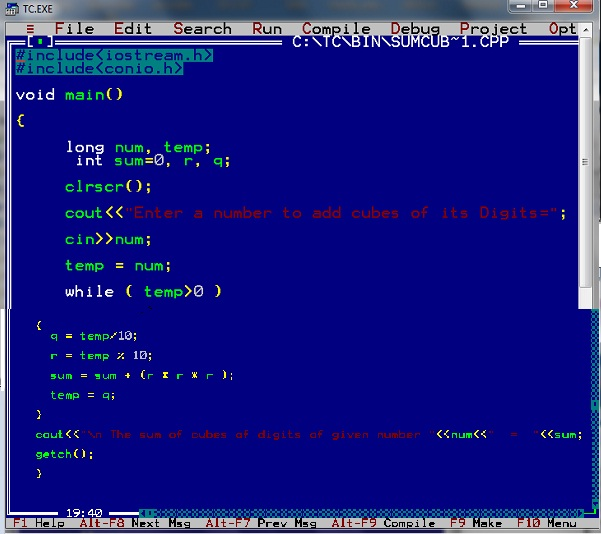# C++ Tutorial With Basic Programs for Beginners

C++ tutorial, C++ programs, C++ code, C++ flowcharts, C++ statements, C++ syntax, C++ examples

## Saturday, March 24, 2018

### C++ Program Compute Sum of Cubes of Digits of User Supplied Number Easyway

#### Q: Write a Program in C++ Programming language that inputs an integer number and computes the sum of cubes of digits of  this user supplied number at run time.

For example, if given number is 123 then answer will be Sum of cubes of  digits = 36
because 13+23+33 = 1+8+27 = 36.C++ Program to compute sum of cubes of digits of user supplied number

### C++ Source Code of Sum of cubes of Digits of a given number Program

/*
Program to input a number, then display sum of cubes of its digits.
(c) Www.EasyCppProgramming.Blogspot.Com
*/
#include<iostream.h>
#include<conio.h>
#include<math.h>
void main()
{
long num, temp;
int, sum=0, r, q;
clrscr();
cout<<"Enter a number to add cubes of its Digits=";
cin>>num;
temp = num;
while ( temp>0 )
{
q = temp/10;
r = temp % 10;
sum = sum +  ( r * r * r);

temp = q;
}
cout<<"\n The sum of cubes of digits of given number "<<num<<"  =  "<<sum;
getch();
}Calculate-cubes-of-digits-of-user-given number

### The Working of  C++ Code of Add Digits of a given number Program

This program is of reverse number logic.

1. We will divide 123 by 10 and get quotient=12, r = 3
2. add r*r*r=3*3*3  into sum, so sum=27

3. Now put q =12 into dividend number
4. so divide 12 by 10, quotient=1 and remainder r=2.
5. Add r*r*r=2*2*2 into sum, so sum will become 27+8 =35.
6. now put q=1 into dividend number

7. Now divide 1 by 10, quotient=0 and r=1.
8. so add r*r*r=1*1*1 into sum, and sum becomes 35+1= 36
9. now put quotient=0 into dividend number called temp variable
10. so that while loop test condition while (temp>0) becomes false and loop terminates.

11. Therefore display the answer sum of cubes of digits of number 123 = 36

Share:

#### Post a Comment

We Love To Hear From You!